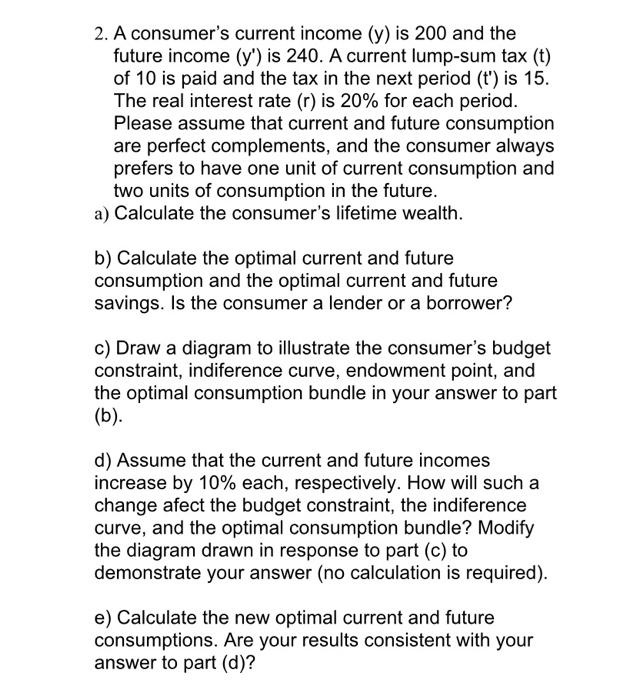Home / Expert Answers / Economics / 2-a-consumer-39-s-current-income-y-is-200-and-the-future-income-left-y-prime-right-pa227

# (Solved): 2. A consumer's current income $$(y)$$ is 200 and the future income $$\left(y^{\prime}\right)$$ ...2. A consumer's current income $$(y)$$ is 200 and the future income $$\left(y^{\prime}\right)$$ is 240 . A current lump-sum tax (t) of 10 is paid and the tax in the next period (t') is 15. The real interest rate $$(r)$$ is $$20 \%$$ for each period. Please assume that current and future consumption are perfect complements, and the consumer always prefers to have one unit of current consumption and two units of consumption in the future. a) Calculate the consumer's lifetime wealth. b) Calculate the optimal current and future consumption and the optimal current and future savings. Is the consumer a lender or a borrower? c) Draw a diagram to illustrate the consumer's budget constraint, indiference curve, endowment point, and the optimal consumption bundle in your answer to part (b). d) Assume that the current and future incomes increase by $$10 \%$$ each, respectively. How will such a change afect the budget constraint, the indiference curve, and the optimal consumption bundle? Modify the diagram drawn in response to part (c) to demonstrate your answer (no calculation is required). e) Calculate the new optimal current and future consumptions. Are your results consistent with your answer to part (d)?

We have an Answer from Expert